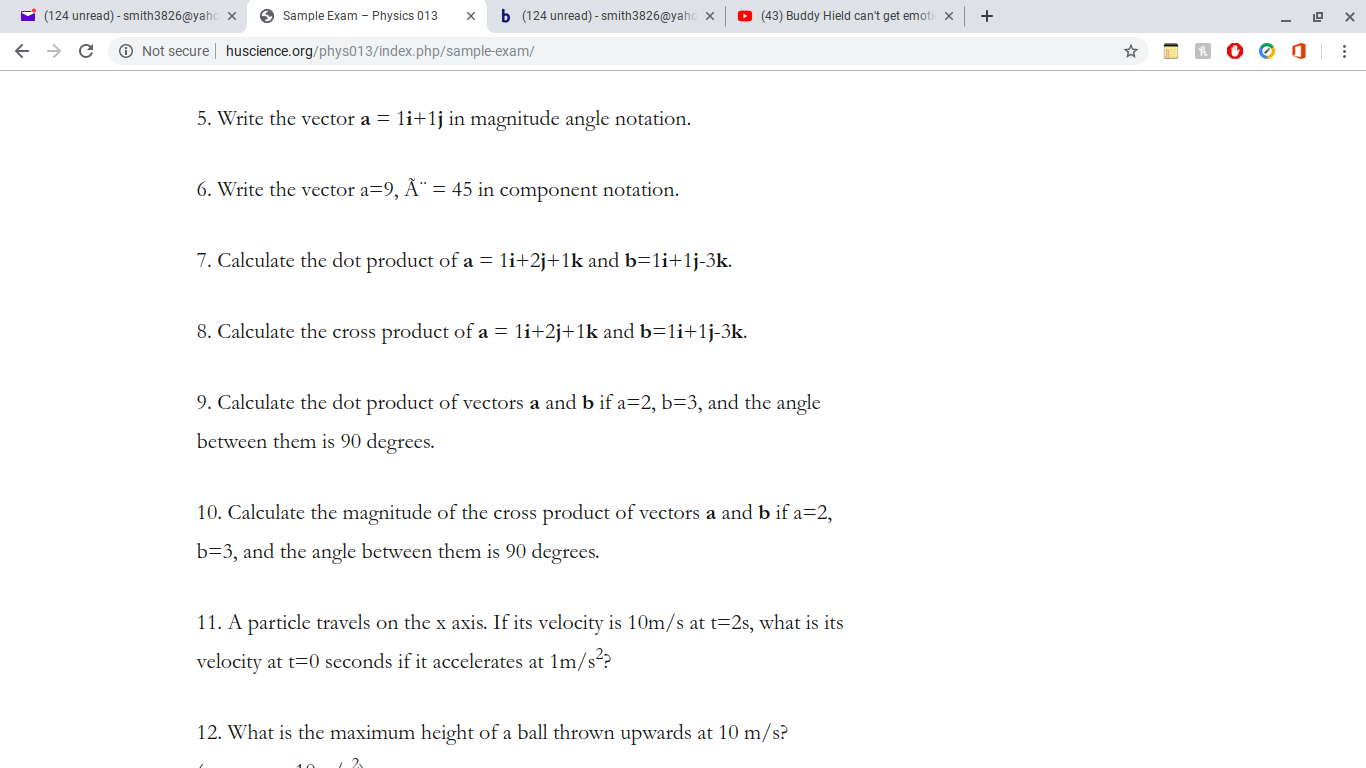# (124 unread) - smith3826@yahoSample ExamPhysics 013b(43) Buddy Hield can't get emotix(124 unread)- smith3826@yahoNot secure huscience.org/phys013/index.php/sample-exam/C5. Write the vector a = li+1j in magnitude angle notation.6. Write the vector a=9, A" = 45 in component notation.7. Calculate the dot product of a li+2j+1k and b 1i+1j-3k.8. Calculate the cross product of a = li+2j+1k and b=1i+1j-3k.9. Calculate the dot product of vcctors a and b if a=2, b-3, and the anglebetween them is 90 degrees.10. Calculate the magnitude of the cross product of vectors a and b if a=2,b-3, and the angle between them is 90 degrees.11. A particle travels on the x axis. If its velocity is 10m/s at t 2s, what is itsvelocity at t0 seconds if it accelerates at 1m/s??12. What is the maximum height of a ball thrown upwards at 10 m/s?

Question

9 and 10. Thanks.help_outlineImage Transcriptionclose(124 unread) - smith3826@yaho Sample Exam Physics 013 b (43) Buddy Hield can't get emotix (124 unread)- smith3826@yaho Not secure huscience.org/phys013/index.php/sample-exam/ C 5. Write the vector a = li+1j in magnitude angle notation. 6. Write the vector a=9, A" = 45 in component notation. 7. Calculate the dot product of a li+2j+1k and b 1i+1j-3k. 8. Calculate the cross product of a = li+2j+1k and b=1i+1j-3k. 9. Calculate the dot product of vcctors a and b if a=2, b-3, and the angle between them is 90 degrees. 10. Calculate the magnitude of the cross product of vectors a and b if a=2, b-3, and the angle between them is 90 degrees. 11. A particle travels on the x axis. If its velocity is 10m/s at t 2s, what is its velocity at t0 seconds if it accelerates at 1m/s?? 12. What is the maximum height of a ball thrown upwards at 10 m/s? fullscreen
check_circleExpert Solution
Step 1

9.

The equation for dot product

Step 2

Substitute a=2 and b=3 and angle 90̊

Step 3

8.

The equation for sca...

### Want to see the full answer?

See Solution

#### Want to see this answer and more?

Solutions are written by subject experts who are available 24/7. Questions are typically answered within 1 hour*

See Solution
*Response times may vary by subject and question
Tagged in

### Vectors and Scalars